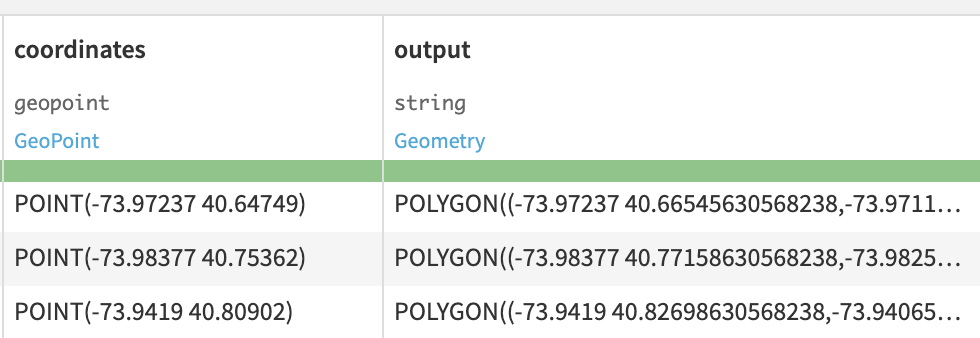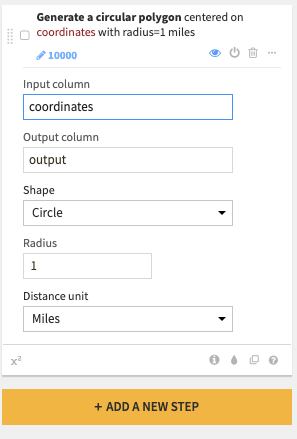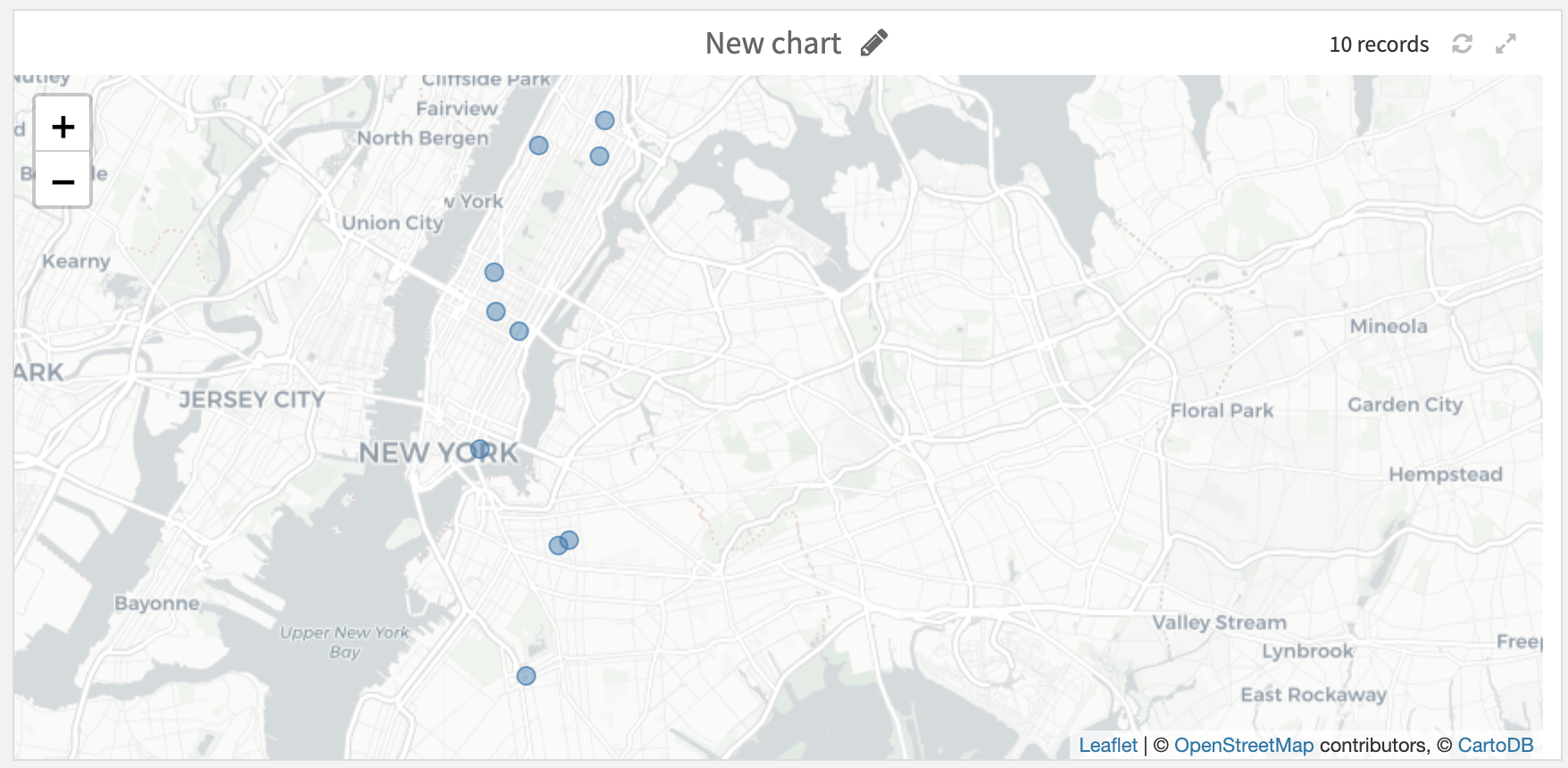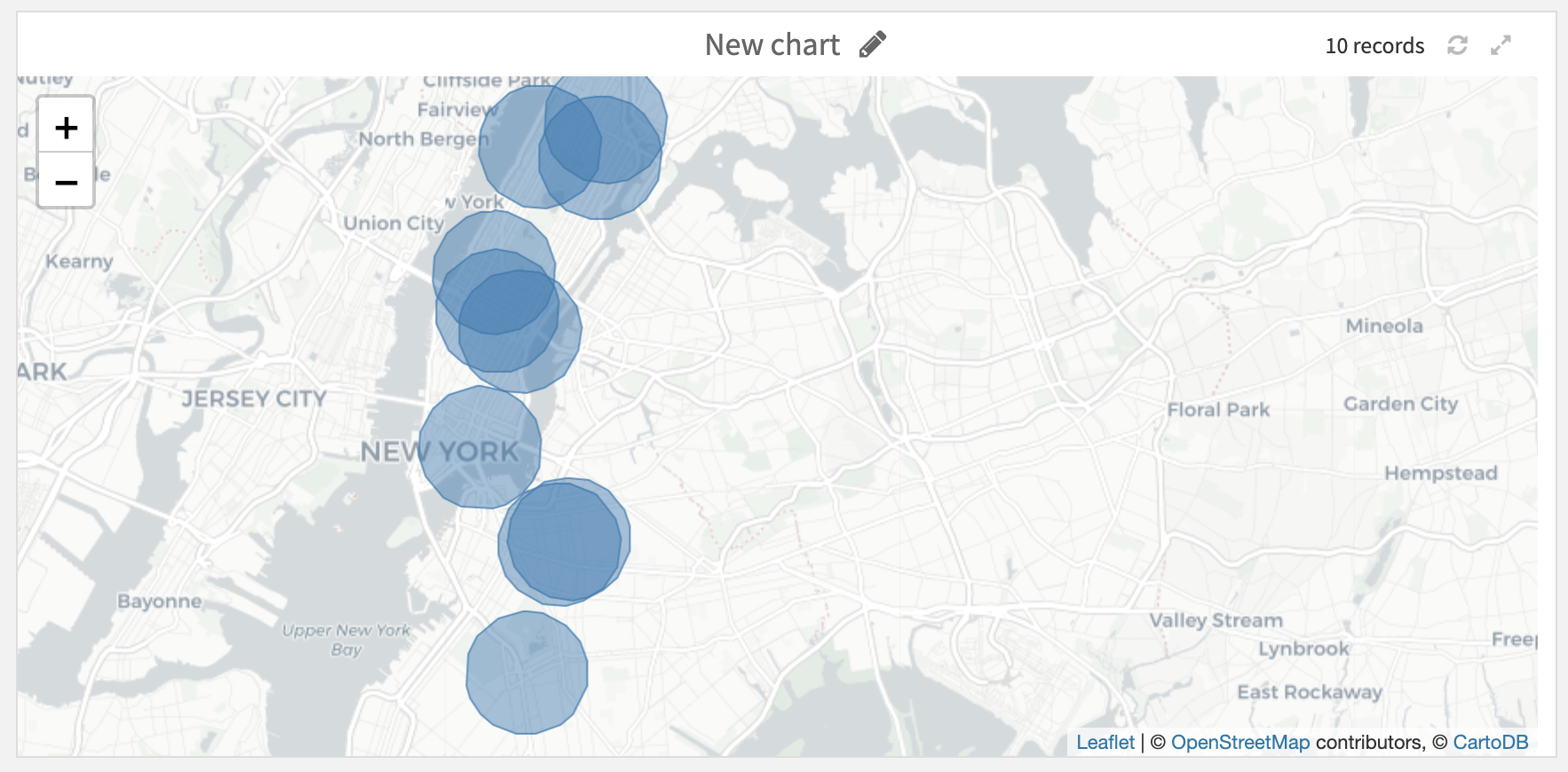# Create area around a geopoint¶

This processor creates buffer polygons around geopoints. For each input geospatial point, a spatial polygon is created around it, delimiting the area of influence covered by the point (all the points that fall within a given distance from the geopoint). The shape area of the polygon can be either rectangular or circular (using an approximation) and the size will depend on the selected parameters.

## Action¶

You can select an input column that contains geopoints on which the polygon is centered. The output column will contain created polygons in the WKT format.

## Polygon creation options¶

Select the shape of polygons from:

• Rectangular

• Circular

Select the unit of distances from:

• Kilometers

• Miles

If Rectangle shape is selected:

• Select the Width and Height of the Rectangle shape to compute.

If Circle shape is selected:

• Select the Radius of the Circle shape to compute.

Each distance is expressed according to the input unit.

## Screenshots¶

Explore view of input and output columns:Parameters of the processor:Input and associated output on a map (Circular shape):